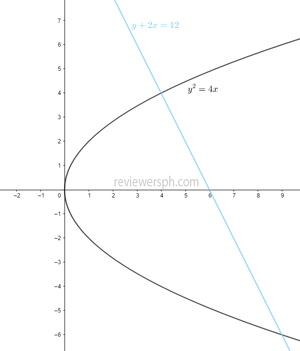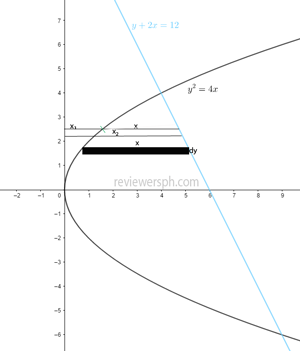### Math Notes

Subjects

#### Integral Calculus Solutions

##### Topics || Problems

Find the area bounded by $$y^2=4x$$ and $$y+2x=12$$
Solution
##### I. Graph the curves.##### II. Calculate the intersections between curves.

$$y=12-2x$$

$$(12-2x)^2 = 4x$$

$$144-48x+4x^2 = 4x$$

$$144-52x+4x^2 = 0$$

$$36-13x+x^2 = 0$$

$$x_1 = 9$$

$$x_2=4$$

Solve the the corresponding values of $$y$$.

At $$x = 9$$

$$y = 12-2(9) = -6$$

At $$x = 4$$

$$y = 12-2(4) = 4$$

##### III. Select the differential rectangle(use horizontal rectangle).The differential area: $$dA=x dy$$ but $$x = x_2-x_1$$

$$x_2=\frac{12-y}{2}$$ : simplified equation of the line

$$x_1 = \frac{y^2}{4}$$

$$dA = [(\frac{12-y}{2})-(\frac{y^2}{4})]dy$$

##### IV. Integrate from the lower limit to the upper limit.

Lower limit:$$y=4$$

Upper limit:$$y=-6$$

$$A = \int_{-6}^4{(\frac{12-y}{2} -\frac{y^2}{4} \,dy )}$$

$$A =6y-\frac{y^2}{4}-\frac{y^3}{12}|_{-6}^4$$

$$A = -(-36-9+18)+(24-4-5.333)$$

$$A = 41.67 \text{sq. units}$$